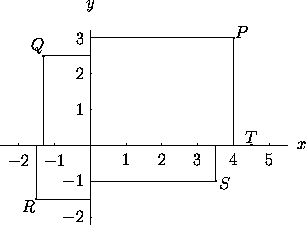# 1.2 Cartesian Coordinates in the Plane

In cartesian coordinates (or rectangular coordinates), the ``address'' of a point P is given by two real numbers indicating the positions of the perpendicular projections from the point to two fixed, perpendicular, graduated lines, called the axes. If one coordinate is denoted x and the other y, the axes are called the x-axis and the y-axis, and we write P=(x,y). Usually the x-axis is drawn horizontal, with x increasing to the right, and the y-axis is drawn vertical, with y increasing going up. The point x=0, y=0 is the origin, where the axes intersect. See Figure 1.Figure 1: In cartesian coordinates, P=(4,3), Q=(-1.3,2.5), R=(-1.5,-1.5), S=(3.5,-1), and T=(4.5,0). The axes divide the plane into four quadrants: P is in the first quadrant, Q in the second, R in the third, and S in the fourth. T is on the positive x-axis.

Next: 1.3 Polar Coordinates in the Plane
Up: 1 Coordinate Systems in the Plane
Previous: 1.1 Substitutions and TransformationsThe Geometry Center Home Page

Silvio Levy
Wed Oct 4 16:41:25 PDT 1995

This document is excerpted from the 30th Edition of the CRC Standard Mathematical Tables and Formulas (CRC Press). Unauthorized duplication is forbidden.